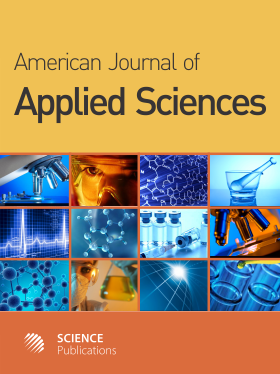Frequency: Continuous
ISSN: 1546-9239 (Print)
ISSN: 1554-3641 (Online)
Research Article Open Access

# Approximation of Iteration Number for Gauss-Seidel Using Redlich-Kister Polynomial

M. K. Hasan, J. Sulaiman, S. Ahmad, M. Othman and S. A.A. Karim

## Abstract

Problem statement: Development of mathematical models based on set of observed data plays a crucial role to describe and predict any phenomena in science, engineering and economics. Therefore, the main purpose of this study was to compare the efficiency of Arithmetic Mean (AM), Geometric Mean (GM) and Explicit Group (EG) iterative methods to solve system of linear equations via estimation of unknown parameters in linear models. Approach: The system of linear equations for linear models generated by using least square method based on (m+1) set of observed data for number of Gauss-Seidel iteration from various grid sizes. Actually there were two types of linear models considered such as piece-wise linear polynomial and piece-wise Redlich-Kister polynomial. All unknown parameters of these models estimated and calculated by using three proposed iterative methods. Results: Thorough several implementations of numerical experiments, the accuracy for formulations of two proposed models had shown that the use of the third-order Redlich-Kister polynomial has high accuracy compared to linear polynomial case. Conclusion: The efficiency of AM and GM iterative methods based on the Redlich-Kister polynomial is superior as compared to EG iterative method.

American Journal of Applied Sciences
Volume 7 No. 7, 2010, 969-975

DOI:

Submitted On: 18 March 2010 Published On: 31 July 2010

How to Cite: Hasan, M. K., Sulaiman, J., Ahmad, S., Othman, M. & Karim, S. A. (2010). Approximation of Iteration Number for Gauss-Seidel Using Redlich-Kister Polynomial. American Journal of Applied Sciences, 7(7), 969-975. https://doi.org/10.3844/ajassp.2010.969.975

• 2,564 Views